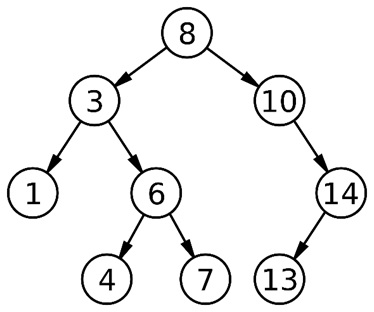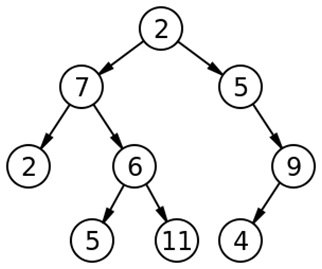Q:

# Check whether a Binary Tree is BST (Binary Search Tree) or not

Given a binary tree check whether it is a binary search tree or not.

Algorithm:

From the definition of BST, it may seem that for a binary tree to be BST, it’s enough to check for each node if the node on its left is smaller & node on its right is greater. But this is actually the wrong approach since it will give wrong output for many test-cases.

The correct algorithm is to check for each node whether the maximum of the left subtree is lesser than the node & the minimum of the right subtree is greater than the node. This algorithm works perfect but not efficient in terms of time complexity.

Intuition says that the in-order traversal for the BST results in a sorted list of nodes and we use this in our algorithm.

```1.  Set prev to INT_MIN.
2.  From main function call checkBST(root, prev)
//passing prev by reference to update it every time
checkBST(root, &prev)
3.  if(root==NULL)
return 1; //null tree is BST
4.  do in-order traversal and checking whether all tree node
data is sorted or not
if(!(checkBST(root->left,prev)))  //check left subtree
return 0;
//root->data must be greater than prevsince BST results in
//sorted list after in-order traversal.
5.  if(root->data<prev)
return 0;
6.  prev=root->data; //update prev value
7.  return checkBST(root->right,prev);//check right subtree```

Example 1:Clearly Example 1 is a binary search tree. We will check out further through our function.

Example 2:Clearly Example 2 is not a binary tree. We will check out through our function.

### C++ class implementation for tree

``````// tree node is defined
class tree{
public:
int data;
tree *left;
tree *right;
};
``````

### C++ function checkBST for implementation

``````//passing reference of prev
int checkBST(tree* root,int &prev){
//null tree is BST
if(root==NULL)
return 1;
//doing inorder traversal and checking whether
//all tree node data is sorted or not
if(!(checkBST(root->left,prev)))
return 0;
if(root->data<prev)
return 0;
prev=root->data; //update prev value

return checkBST(root->right,prev);
}
``````

### C++ implementation for creating tree nodes

``````// creating new node
tree* newnode(int data)
{
tree* node = (tree*)malloc(sizeof(tree));
node->data = data;
node->left = NULL;
node->right = NULL;

return(node);
}
``````

### Main driver function for example1

``````#include <bits/stdc++.h>
using namespace std;

int main()
{
//**same tree is builted as shown in example**
int c,prev=INT_MIN;//prev initialized to INT_MIN
cout<<"Tree is built like the example 1 aforesaid"<<endl;

tree *root=newnode(8);
root->left= newnode(3);
root->right= newnode(10);
root->right->right=newnode(14);
root->right->right->left=newnode(13);
root->left->left=newnode(1);
root->left->right=newnode(6);
root->left->right->left=newnode(4);
root->left->right->right=newnode(7);

cout<<"builting the binary tree like example 1......"<<endl;
c=checkBST(root,prev);
if(c)
cout<<"This binary tree is binary search tree"<<endl;
else
cout<<"This is not a binary search tree";

return 0;
}
``````

### Main driver function for example2

``````#include <bits/stdc++.h>
using namespace std;

int main()
{
//**same tree is builted as shown in example**
int c,prev=INT_MIN;//prev initialized to INT_MIN
cout<<"Tree is built like the example 2 aforesaid"<<endl;

tree *root=newnode(2);
root->left= newnode(7);
root->right= newnode(5);
root->right->right=newnode(9);
root->right->right->left=newnode(4);
root->left->left=newnode(2);
root->left->right=newnode(6);
root->left->right->left=newnode(5);
root->left->right->right=newnode(11);

cout<<"builting the binary tree like example 2......"<<endl;
c=checkBST(root,prev);
if(c)
cout<<"This binary tree is binary search tree"<<endl;
else
cout<<"This is not a binary search tree";

return 0;
}
``````

Output 1

```Tree is built like the example 1 aforesaid
builting the binary tree like example 1......
This binary tree is binary search tree
```

Output 2

```Tree is built like the example 2 aforesaid
builting the binary tree like example 2......
This is not a binary search tree ```